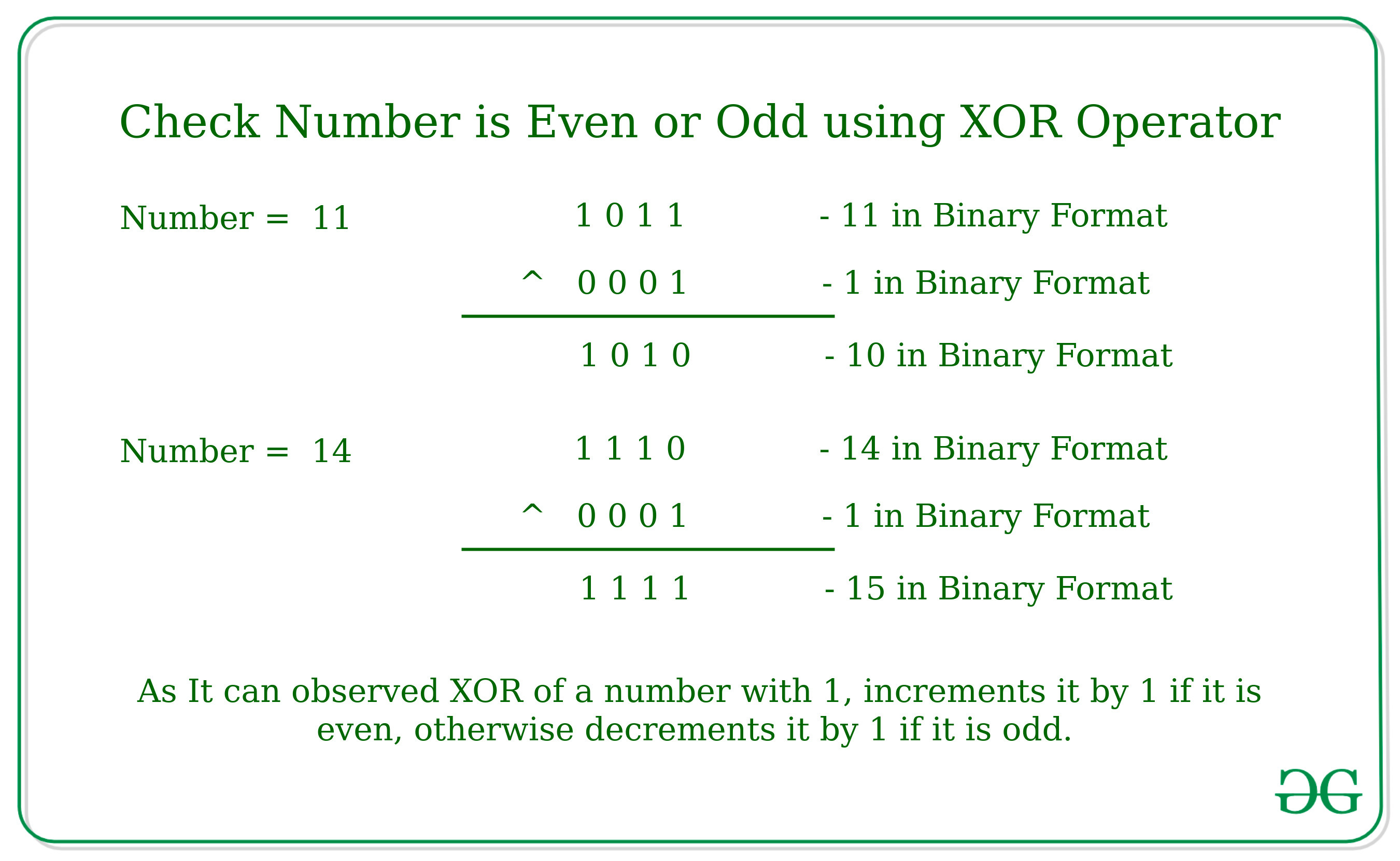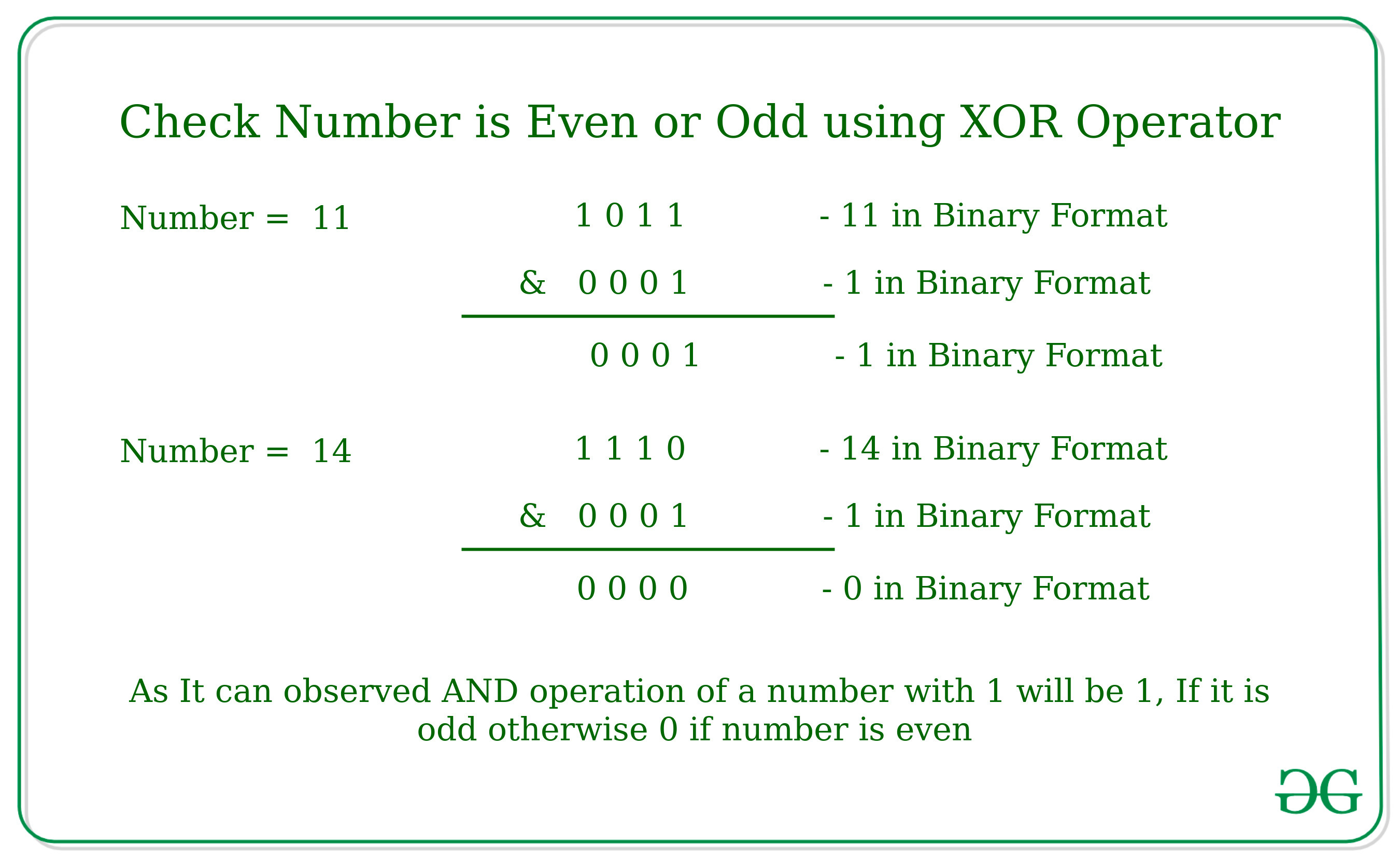# Check if a Number is Odd or Even using Bitwise Operators

Given a number N, the task is to check whether the number is even or odd using Bitwise Operators.

Examples:

Input: N = 11
Output: Odd

Input: N = 10
Output: Even

## Recommended: Please try your approach on {IDE} first, before moving on to the solution.

Following Bitwise Operators can be used to check if a number is odd or even:

1. Using Bitwise XOR operator:
The idea is to check whether last bit of the number is set or not. If last bit is set then the number is odd, otherwise even.

As we know bitwise XOR Operation of the Number by 1 increment the value of the number by 1 if the number is even otherwise it decrements the value of the number by 1 if the value is odd.Below is the implementation of the above approach:

## C++

 `// C++ program to check for even or odd ` `// using Bitwise XOR operator ` ` `  `#include ` `using` `namespace` `std; ` ` `  `// Returns true if n is even, else odd ` `bool` `isEven(``int` `n) ` `{ ` ` `  `    ``// n^1 is n+1, then even, else odd ` `    ``if` `(n ^ 1 == n + 1) ` `        ``return` `true``; ` `    ``else` `        ``return` `false``; ` `} ` ` `  `// Driver code ` `int` `main() ` `{ ` `    ``int` `n = 100; ` `    ``isEven(n)  ` `? cout << ``"Even"`  `: cout << ``"Odd"``; ` ` `  `    ``return` `0; ` `} `

## Java

 `// Java program to check for even or odd ` `// using Bitwise XOR operator ` `class` `GFG ` `{ ` ` `  `    ``// Returns true if n is even, else odd ` `    ``static` `boolean` `isEven(``int` `n) ` `    ``{ ` ` `  `        ``// n^1 is n+1, then even, else odd ` `        ``if` `((n ^ ``1``) == n + ``1``) ` `            ``return` `true``; ` `        ``else` `            ``return` `false``; ` `    ``} ` ` `  `    ``// Driver code ` `    ``public` `static` `void` `main(String[] args) ` `    ``{ ` `        ``int` `n = ``100``; ` `        ``System.out.print(isEven(n) == ``true` `? ``"Even"` `: ``"Odd"``); ` `    ``} ` `} ` ` `  `// This code is contributed by Rajput-Ji `

## Python3

 `# Python3 program to check for even or odd  ` `# using Bitwise XOR operator  ` ` `  `# Returns true if n is even, else odd  ` `def` `isEven( n) :  ` ` `  `    ``# n^1 is n+1, then even, else odd  ` `    ``if` `(n ^ ``1` `=``=` `n ``+` `1``) : ` `        ``return` `True``;  ` `    ``else` `: ` `        ``return` `False``;  ` ` `  `# Driver code  ` `if` `__name__ ``=``=` `"__main__"` `:  ` `    ``n ``=` `100``;  ` `    ``print``(``"Even"``) ``if` `isEven(n) ``else` `print``( ``"Odd"``);  ` ` `  `# This code is contributed by AnkitRai01 `

## C#

 `// C# program to check for even or odd ` `// using Bitwise XOR operator ` `using` `System; ` ` `  `class` `GFG ` `{ ` ` `  `    ``// Returns true if n is even, else odd ` `    ``static` `bool` `isEven(``int` `n) ` `    ``{ ` ` `  `        ``// n^1 is n+1, then even, else odd ` `        ``if` `((n ^ 1) == n + 1) ` `            ``return` `true``; ` `        ``else` `            ``return` `false``; ` `    ``} ` ` `  `    ``// Driver code ` `    ``public` `static` `void` `Main(String[] args) ` `    ``{ ` `        ``int` `n = 100; ` `        ``Console.Write(isEven(n) == ``true` `? ``"Even"` `: ``"Odd"``); ` `    ``} ` `} ` ` `  `// This code is contributed by Rajput-Ji `

Output :

```Even
```
2. Using Bitwise AND operator:
The idea is to check whether the last bit of the number is set or not. If last bit is set then the number is odd, otherwise even.

As we know bitwise AND Operation of the Number by 1 will be 1, If it is odd because the last bit will be already set. Otherwise it will give 0 as output.Below is the implementation of the above approach:

## C++

 `// C++ program to check for even or odd ` `// using Bitwise AND operator ` ` `  `#include   ` `using` `namespace` `std;  ` `   `  `// Returns true if n is even, else odd  ` `bool` `isEven(``int` `n)  ` `{  ` `    ``// n&1 is 1, then odd, else even  ` `    ``return` `(!(n & 1));  ` `}  ` `   `  `// Driver code  ` `int` `main()  ` `{  ` `    ``int` `n = 101;  ` `    ``isEven(n)  ` `? cout << ``"Even"`  `: cout << ``"Odd"``;  ` `    ``return` `0;  ` `}  `

## Java

 `// Java program to check for even or odd ` `// using Bitwise AND operator ` `class` `GFG ` `{  ` `     `  `// Returns true if n is even, else odd  ` `static` `boolean` `isEven(``int` `n)  ` `{  ` `    ``// n&1 is 1, then odd, else even  ` `    ``return` `((n & ``1``)!=``1``);  ` `}  ` `     `  `// Driver code  ` `public` `static` `void` `main(String[] args)  ` `{  ` `    ``int` `n = ``101``;  ` `    ``System.out.print(isEven(n) == ``true` `? ``"Even"` `: ``"Odd"``);  ` `}  ` `}  ` ` `  `// This code is contributed by PrinciRaj1992 `

## Python3

 `# Python3 program to check for even or odd ` `# using Bitwise AND operator ` ` `  `# Returns true if n is even, else odd  ` `def` `isEven(n) :  ` ` `  `    ``# n&1 is 1, then odd, else even  ` `    ``return` `(``not``(n & ``1``));  ` ` `  `# Driver code  ` `if` `__name__ ``=``=` `"__main__"` `:  ` ` `  `    ``n ``=` `101``;  ` `    ``if` `isEven(n) : ` `        ``print``(``"Even"``) ` `    ``else``: ` `        ``print``(``"Odd"``);  ` ` `  `# This code is contributed by AnkitRai01 `

## C#

 `// C# program to check for even or odd ` `// using Bitwise AND operator ` `using` `System; ` ` `  `class` `GFG ` `{  ` `     `  `    ``// Returns true if n is even, else odd  ` `    ``static` `bool` `isEven(``int` `n)  ` `    ``{  ` `        ``// n&1 is 1, then odd, else even  ` `        ``return` `((n & 1) != 1);  ` `    ``}  ` `         `  `    ``// Driver code  ` `    ``public` `static` `void` `Main()  ` `    ``{  ` `        ``int` `n = 101;  ` `        ``Console.Write(isEven(n) == ``true` `? ``"Even"` `: ``"Odd"``);  ` `    ``}  ` `}  ` ` `  `// This code is contributed by AnkitRai01 `

Output:

```Odd
```

Don’t stop now and take your learning to the next level. Learn all the important concepts of Data Structures and Algorithms with the help of the most trusted course: DSA Self Paced. Become industry ready at a student-friendly price.

My Personal Notes arrow_drop_upCheck out this Author's contributed articles.

If you like GeeksforGeeks and would like to contribute, you can also write an article using contribute.geeksforgeeks.org or mail your article to contribute@geeksforgeeks.org. See your article appearing on the GeeksforGeeks main page and help other Geeks.

Please Improve this article if you find anything incorrect by clicking on the "Improve Article" button below.

Article Tags :
Practice Tags :

2

Please write to us at contribute@geeksforgeeks.org to report any issue with the above content.